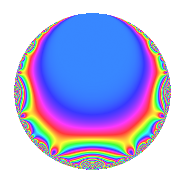# Properties

 Label 6034.2.a.iLevel 6034 Weight 2 Character orbit 6034.a Self dual Yes Analytic conductor 48.182 Analytic rank 0 Dimension 2 CM No Inner twists 1

# Related objects

## Newspace parameters

 Level: $$N$$ = $$6034 = 2 \cdot 7 \cdot 431$$ Weight: $$k$$ = $$2$$ Character orbit: $$[\chi]$$ = 6034.a (trivial)

## Newform invariants

 Self dual: Yes Analytic conductor: $$48.1817325796$$ Analytic rank: $$0$$ Dimension: $$2$$ Coefficient field: $$\Q(\sqrt{3})$$ Coefficient ring: $$\Z[a_1, \ldots, a_{13}]$$ Coefficient ring index: $$1$$ Fricke sign: $$-1$$ Sato-Tate group: $\mathrm{SU}(2)$

## $q$-expansion

Coefficients of the $$q$$-expansion are expressed in terms of $$\beta = \sqrt{3}$$. We also show the integral $$q$$-expansion of the trace form.

 $$f(q)$$ $$=$$ $$q + q^{2} + 2 q^{3} + q^{4} + 2 q^{6} + q^{7} + q^{8} + q^{9} +O(q^{10})$$ $$q + q^{2} + 2 q^{3} + q^{4} + 2 q^{6} + q^{7} + q^{8} + q^{9} + ( 2 - 2 \beta ) q^{11} + 2 q^{12} + ( -3 + \beta ) q^{13} + q^{14} + q^{16} -2 \beta q^{17} + q^{18} + ( 4 + 2 \beta ) q^{19} + 2 q^{21} + ( 2 - 2 \beta ) q^{22} + 6 q^{23} + 2 q^{24} -5 q^{25} + ( -3 + \beta ) q^{26} -4 q^{27} + q^{28} + ( 4 - 2 \beta ) q^{29} + ( 2 + 2 \beta ) q^{31} + q^{32} + ( 4 - 4 \beta ) q^{33} -2 \beta q^{34} + q^{36} + ( 7 + \beta ) q^{37} + ( 4 + 2 \beta ) q^{38} + ( -6 + 2 \beta ) q^{39} + 4 \beta q^{41} + 2 q^{42} + ( 3 - 5 \beta ) q^{43} + ( 2 - 2 \beta ) q^{44} + 6 q^{46} + ( 2 + 6 \beta ) q^{47} + 2 q^{48} + q^{49} -5 q^{50} -4 \beta q^{51} + ( -3 + \beta ) q^{52} + 2 q^{53} -4 q^{54} + q^{56} + ( 8 + 4 \beta ) q^{57} + ( 4 - 2 \beta ) q^{58} + 2 \beta q^{59} + ( 2 + 2 \beta ) q^{61} + ( 2 + 2 \beta ) q^{62} + q^{63} + q^{64} + ( 4 - 4 \beta ) q^{66} + ( -1 - \beta ) q^{67} -2 \beta q^{68} + 12 q^{69} + ( 4 + 4 \beta ) q^{71} + q^{72} + 2 q^{73} + ( 7 + \beta ) q^{74} -10 q^{75} + ( 4 + 2 \beta ) q^{76} + ( 2 - 2 \beta ) q^{77} + ( -6 + 2 \beta ) q^{78} + 12 q^{79} -11 q^{81} + 4 \beta q^{82} + ( -3 - 5 \beta ) q^{83} + 2 q^{84} + ( 3 - 5 \beta ) q^{86} + ( 8 - 4 \beta ) q^{87} + ( 2 - 2 \beta ) q^{88} -2 q^{89} + ( -3 + \beta ) q^{91} + 6 q^{92} + ( 4 + 4 \beta ) q^{93} + ( 2 + 6 \beta ) q^{94} + 2 q^{96} -8 q^{97} + q^{98} + ( 2 - 2 \beta ) q^{99} +O(q^{100})$$ $$\operatorname{Tr}(f)(q)$$ $$=$$ $$2q + 2q^{2} + 4q^{3} + 2q^{4} + 4q^{6} + 2q^{7} + 2q^{8} + 2q^{9} + O(q^{10})$$ $$2q + 2q^{2} + 4q^{3} + 2q^{4} + 4q^{6} + 2q^{7} + 2q^{8} + 2q^{9} + 4q^{11} + 4q^{12} - 6q^{13} + 2q^{14} + 2q^{16} + 2q^{18} + 8q^{19} + 4q^{21} + 4q^{22} + 12q^{23} + 4q^{24} - 10q^{25} - 6q^{26} - 8q^{27} + 2q^{28} + 8q^{29} + 4q^{31} + 2q^{32} + 8q^{33} + 2q^{36} + 14q^{37} + 8q^{38} - 12q^{39} + 4q^{42} + 6q^{43} + 4q^{44} + 12q^{46} + 4q^{47} + 4q^{48} + 2q^{49} - 10q^{50} - 6q^{52} + 4q^{53} - 8q^{54} + 2q^{56} + 16q^{57} + 8q^{58} + 4q^{61} + 4q^{62} + 2q^{63} + 2q^{64} + 8q^{66} - 2q^{67} + 24q^{69} + 8q^{71} + 2q^{72} + 4q^{73} + 14q^{74} - 20q^{75} + 8q^{76} + 4q^{77} - 12q^{78} + 24q^{79} - 22q^{81} - 6q^{83} + 4q^{84} + 6q^{86} + 16q^{87} + 4q^{88} - 4q^{89} - 6q^{91} + 12q^{92} + 8q^{93} + 4q^{94} + 4q^{96} - 16q^{97} + 2q^{98} + 4q^{99} + O(q^{100})$$

## Embeddings

For each embedding $$\iota_m$$ of the coefficient field, the values $$\iota_m(a_n)$$ are shown below.

For more information on an embedded modular form you can click on its label.

Label $$\iota_m(\nu)$$ $$a_{2}$$ $$a_{3}$$ $$a_{4}$$ $$a_{5}$$ $$a_{6}$$ $$a_{7}$$ $$a_{8}$$ $$a_{9}$$ $$a_{10}$$
1.1
 1.73205 −1.73205
1.00000 2.00000 1.00000 0 2.00000 1.00000 1.00000 1.00000 0
1.2 1.00000 2.00000 1.00000 0 2.00000 1.00000 1.00000 1.00000 0
 $$n$$: e.g. 2-40 or 990-1000 Significant digits: Format: Complex embeddings Normalized embeddings Satake parameters Satake angles

## Inner twists

This newform does not admit any (nontrivial) inner twists.

## Atkin-Lehner signs

$$p$$ Sign
$$2$$ $$-1$$
$$7$$ $$-1$$
$$431$$ $$-1$$

## Hecke kernels

This newform can be constructed as the intersection of the kernels of the following linear operators acting on $$S_{2}^{\mathrm{new}}(\Gamma_0(6034))$$:

 $$T_{3} - 2$$ $$T_{5}$$ $$T_{11}^{2} - 4 T_{11} - 8$$